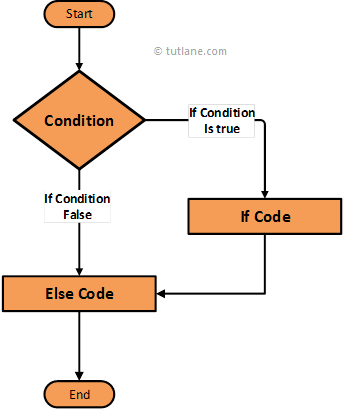# Swift If Else Statement

In swift, the if-else statement is useful to add an alternative set of else statements for if condition. In case if condition fails (FALSE) then alternatively it will execute else statements.

Whenever we have specific requirements like executing a set of block statements if given condition is TRUE else execute other statements in that situation we can use swift if-else statement.

Now we will see the functionality of if-else statement in a swift programming language using an algorithm diagram.

## Swift If Else Statement Flow Diagram

Following is the swift if-else statement flow diagram to represent how the functionality of if-else statement will work in a swift programming language.If you observe the above swift if-else statement flow diagram first it will evaluate the given condition is TRUE or FALSE. If the given condition is TRUE then it will execute the code block within if statement. In case the given condition is FALSE then it will execute else condition code block and reach the end of the statement.

## Syntax of Swift If Else Statement

Following is the syntax of an if-else statement in swift programming language.

If condition

{

//Expressions will execute when condition TRUE

}

else

{

//Expressions will execute when if the condition fails

}

If you observe the above swift if-else statement syntax first it will evaluate if condition if it TRUE then it will execute if condition expression otherwise it will execute else condition expressions.

Now we will see how to use if-else statement in a swift programming language with examples.

## Swift If Else Statement Example

Following is the simple example using the if-else statement in swift to check the condition before executing the expressions within the condition.

var num1: Int = 10

if num1 > 20 {

print("Number Greater than 20")

}

else {

print("Number Less than 20 ")

}

If you observe above example we are checking the condition whether the value of “num1” greater 20 or not.

When we execute the above code in the swift playground we will get a result like as shown below.

Number Less than 20

This is how we can use swift if-else statements to execute expressions based on our defined conditions.